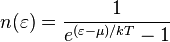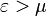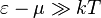# Bose-Einstein statistics facts for kids

Kids Encyclopedia Facts

In statistical mechanics, Bose-Einstein statistics means the statistics of a system where you can not tell the difference between any of the particles, and the particles are bosons. Bosons are fundamental particles like the photon.

The Bose-Einstein distribution tells you how many particles have a certain energy. The formula is$n(\varepsilon) = \frac{1}{e^{(\varepsilon-\mu)/kT}-1}$

with$\varepsilon > \mu$ and where:

n(ε)  is the number of particles which have energy ε
ε  is the energy
μ is the chemical potential
k is Boltzmann's constant
T is the temperature

If$\varepsilon-\mu \gg kT$, then the Maxwell–Boltzmann statistics is a good approximation.Bose-Einstein statistics Facts for Kids. Kiddle Encyclopedia.